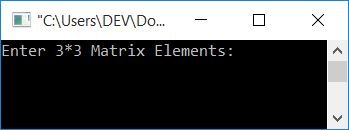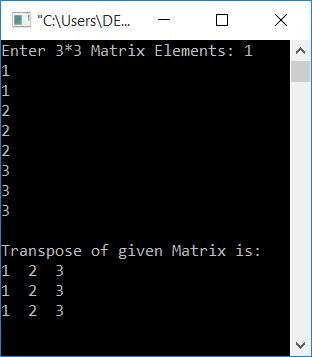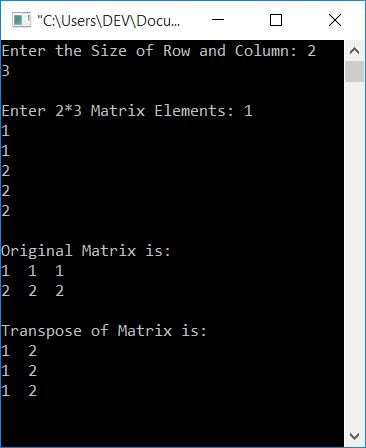# C Program to Find the Transpose of a Matrix

In this article, you will learn and get code to find the transpose of a given matrix by the user at run-time using a C program. But before starting the program, let's first understand how to find the transpose of any matrix.

### How to Find the Transpose of a Matrix

A matrix's transpose can be calculated by swapping rows for columns or columns for rows. For example, if a given matrix, say Matrix A, is:

```1  1  1
2  2  2
3  3  3```

Then, its transpose, say AT, will be:

```1  2  3
1  2  3
1  2  3```

As you can see, all three rows get switched to three columns in a way that:

• The element in the 0th row and 0th column of the original matrix is transferred to the 0th row and 0th column of AT.
• The element in the 0th row and 1st column of the original matrix is transferred to the 1st row and 0th column of AT.
• The element in the 0th row and 2nd column of the original matrix is transferred to the 2nd row and 0th column of AT.
• The element in the 1st row and 0th column of the original matrix is transferred to the 0th row and 1st column of AT.
• The element in the 1st row and 1st column of the original matrix is transferred to the 1st row and 1st column of AT.
• The element in the 1st row and 2nd column of the original matrix is transferred to the 2nd row and 1st column of AT.
• In a similar way, the next three elements of the original matrix go to matrix AT.

## In C, find the transpose of a matrix

This program is created for a 3x3 matrix. That is a matrix with 3 rows and 3 columns. Therefore, this program asks the user to enter nine elements for the matrix and then finds its transpose:

```#include<stdio.h>
#include<conio.h>
int main()
{
int mat, i, j, matTrans;
printf("Enter 3*3 Matrix Elements: ");
for(i=0; i<3; i++)
{
for(j=0; j<3; j++)
scanf("%d", &mat[i][j]);
}
// Transposing the Matrix...
for(i=0; i<3; i++)
{
for(j=0; j<3; j++)
matTrans[j][i] = mat[i][j];
}
printf("\nTranspose of given Matrix is:\n");
for(i=0; i<3; i++)
{
for(j=0; j<3; j++)
printf("%d  ", matTrans[i][j]);
printf("\n");
}
getch();
return 0;
}```

This program was built and runs in the Code::Blocks IDE. Here is its sample run:Now enter any nine elements for the matrix and press the ENTER key to see the following output:The following block of code is responsible for transposing the matrix:

```for(i=0; i<3; i++)
{
for(j=0; j<3; j++)
matTrans[j][i] = mat[i][j];
}```

If you want to learn about for loops in detail, then refer to the separate tutorial on it. But for now, the above code block executes in a way that the initialization part, say i = 0, executes only once at the start of the loop, and every time while entering the block of code inside the loop, its condition (the second statement of the loop) must be checked. If the condition evaluates to true, then only the program flow goes inside the body of the loop and executes all the statements.

Assume the user enters nine elements as 1, 1, 1, 2, 2, 3, 3. Therefore, mat=1, mat=1, mat=1, mat=2, mat=2, and so on. Because i starts at 0 and executes until its value becomes 3, the loop executes a total of 3 times. That is, both the loops execute three times in such a way that:

• At the first execution of the outer for loop, the inner for loop executes three times. Therefore
• During the first execution, `matTrans[j][i] = mat[i][j]` or `matTrans = mat` or matTrans = 1.
• During the second execution, `matTrans[j][i] = mat[i][j]` or `matTrans = mat` or matTrans = 1.
• During the third execution, `matTrans[j][i] = mat[i][j]` or `matTrans = mat` or matTrans = 1.
• At the second execution of the outer for loop, the inner for loop again executes three times. Therefore
• During the first execution, `matTrans[j][i] = mat[i][j]` or `matTrans = mat` or matTrans = 2.
• During the second execution, `matTrans[j][i] = mat[i][j]` or `matTrans = mat` or matTrans = 2.
• During the third execution, `matTrans[j][i] = mat[i][j]` or `matTrans = mat` or matTrans = 2.
• The inner for loop is executed three times after the third execution of the outer for loop. Therefore
• During the first execution, `matTrans[j][i] = mat[i][j]` or `matTrans = mat` or matTrans = 3.
• During the second execution, `matTrans[j][i] = mat[i][j]` or `matTrans = mat` or matTrans = 3.
• During the third execution, `matTrans[j][i] = mat[i][j]` or `matTrans = mat` or matTrans = 3.

As a result, we will have a matrix named matTrans[][] with the value:

• matTrans = 1
• matTrans = 2
• matTrans = 3
• matTrans = 1
• matTrans = 2
• matTrans = 2
• matTrans = 1
• matTrans = 2
• matTrans = 3

which is the transpose of the given matrix A.

### Allow the user to specify the dimensions of the matrix

This program allows the user to define the dimensions of the original matrix. That is, the user has to enter the size of the rows and columns for the matrix. And also enter the matrix element of the given size. Then it will calculate and print the transpose.

```#include<stdio.h>
#include<conio.h>
int main()
{
int mat, rowSize, colSize, i, j, matTrans;
printf("Enter the Size of Row and Column: ");
scanf("%d%d", &rowSize, &colSize);
printf("\nEnter %d*%d Matrix Elements: ", rowSize, colSize);
for(i=0; i<rowSize; i++)
{
for(j=0; j<colSize; j++)
scanf("%d", &mat[i][j]);
}
printf("\nOriginal Matrix is:\n");
for(i=0; i<rowSize; i++)
{
for(j=0; j<colSize; j++)
printf("%d  ", mat[i][j]);
printf("\n");
}
// Transposing the Matrix.....
for(i=0; i<rowSize; i++)
{
for(j=0; j<colSize; j++)
matTrans[j][i] = mat[i][j];
}
printf("\nTranspose of Matrix is:\n");
for(i=0; i<colSize; i++)
{
for(j=0; j<rowSize; j++)
printf("%d  ", matTrans[i][j]);
printf("\n");
}
getch();
return 0;
}```

Here is it sample run:#### The same program in different languages

C Quiz

« Previous Program Next Program »